﻿ 基于深度森林的卫星ACS执行机构与传感器故障识别Download PDF文章快速检索 高级检索

1. 南京航空航天大学 自动化学院, 南京 211100;
2. 南京航空航天大学 航天学院, 南京 210016

Fault identification of actuators and sensors of satellite attitude control systems based on deep forest algorithm
CHENG Yuehua1, JIANG Wenjian1, YANG Hao1, XUE Qi1, LIAO He2
1. School of Automation Engineering, Nanjing University of Aeronautics and Astronautics, Nanjing 211100, China;
2. School of Astronautics, Nanjing University of Aeronautics and Astronautics, Nanjing 210016, China
Abstract: The difficulty in identifying the faulted sensors and actuators of satellite Attitude Control Systems (ACS) resides in the spreading of faults in the closed loop. The deep forest algorithm is introduced in this study to build a fault prediction model to achieve the isolation of the sensor faults and actuator faults. After collecting healthy ACS telemetry data to group a training set according to the dynamic characteristics of ACS, we apply appropriate feature selection and extraction methods to the training set, obtaining the features of both the sensor faults and actuator faults. The deep forest algorithm, with its strong generalization ability, is then used to learn and identify fault information, thereby establishing a fault prediction model to realize the recognition of actuator and sensor faults. The results of the semi-physical simulation indicate that the proposed method can identify the faults of sensors and actuators effectively in the presence of many uncertain factors, such as the interference moments of air bearing testbeds, unknown moments of the inertia of satellites, nonlinear characteristics of flywheels and fault propagation in the closed loop of ACS.
Keywords: deep forest algorithm    satellite attitude control systems    actuators    sensors    fault identification

1 问题的提出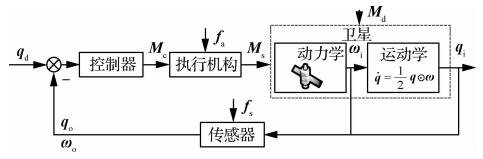图 1 卫星ACS结构框图 Fig. 1 Structure diagram of satellite attitude control systems

 $\mathit{\boldsymbol{\dot q = }}\frac{1}{2}\mathit{\boldsymbol{q}} \odot \mathit{\boldsymbol{\omega = }}\frac{1}{2}\mathit{\boldsymbol{ \boldsymbol{\varXi} }}(\mathit{\boldsymbol{q}})\mathit{\boldsymbol{\omega }}$ （1）

 $[\mathit{\boldsymbol{q}} \odot ]\mathit{\boldsymbol{ = }}\left[ {\begin{array}{*{20}{c}} {{\mathit{\boldsymbol{q}}_4}{\mathit{\boldsymbol{I}}_3} + [{\mathit{\boldsymbol{q}}_{1:3}} \times ]}&{{\mathit{\boldsymbol{q}}_{1:3}}}\\ { - \mathit{\boldsymbol{q}}_{1:3}^{\rm{T}}}&{{\mathit{\boldsymbol{q}}_4}} \end{array}} \right] = [\mathit{\boldsymbol{ \boldsymbol{\varXi} }}(\mathit{\boldsymbol{q}})\;\;\mathit{\boldsymbol{q}}]$ （2）
 $\mathit{\boldsymbol{ \boldsymbol{\varXi} }}(\mathit{\boldsymbol{q}}) = \left[ {\begin{array}{*{20}{c}} {{\mathit{\boldsymbol{q}}_4}{\mathit{\boldsymbol{I}}_3} + [{\mathit{\boldsymbol{q}}_{1:3}} \times ]}\\ { - \mathit{\boldsymbol{q}}_{1:3}^{\rm{T}}} \end{array}} \right] = \left[ {\begin{array}{*{20}{c}} {{\mathit{\boldsymbol{q}}_4}}&{ - {\mathit{\boldsymbol{q}}_3}}&{{\mathit{\boldsymbol{q}}_2}}\\ {{\mathit{\boldsymbol{q}}_3}}&{{\mathit{\boldsymbol{q}}_4}}&{ - {\mathit{\boldsymbol{q}}_1}}\\ { - {\mathit{\boldsymbol{q}}_2}}&{{\mathit{\boldsymbol{q}}_1}}&{{\mathit{\boldsymbol{q}}_4}}\\ { - {\mathit{\boldsymbol{q}}_1}}&{ - {\mathit{\boldsymbol{q}}_2}}&{ - {\mathit{\boldsymbol{q}}_3}} \end{array}} \right]$ （3）

 ${\mathit{\boldsymbol{J}}_{\rm{s}}}\mathit{\boldsymbol{\dot \omega + \omega }} \times ({\mathit{\boldsymbol{J}}_{\rm{s}}}\mathit{\boldsymbol{\omega + }}{\mathit{\boldsymbol{H}}_{\rm{w}}}) = {\mathit{\boldsymbol{M}}_{\rm{c}}} + {\mathit{\boldsymbol{M}}_{\rm{d}}}$ （4）

1) 卫星运行空间环境复杂，难以建立精确的干扰力矩Md模型。干扰动量轮对卫星ACS的控制力矩，从而影响系统输出。

2) 反作用飞轮作为典型的卫星姿态控制部件，已经成为控制系统不可缺少的配置。但由于飞轮在进行姿态调整过程中，非线性摩擦、电机噪声以及轴承噪声等多种未知因素会带来控制中的不确定干扰力矩，难以精确Mδ的模型。

3) 执行机构发生故障时，其表现主要为执行机构的实际输出力矩会产生偏差Mf，通过动力学与运动学方程，影响传感器测量输出ωoqo。而传感器发生故障时，会通过叠加故障值fs，通过ACS闭环影响控制器的指令输出力矩Mc。ACS中执行机构与传感器故障在系统中传播和相互影响，给故障诊断和辨识带来了困难和挑战。

2 卫星ACS执行机构和传感器故障识别策略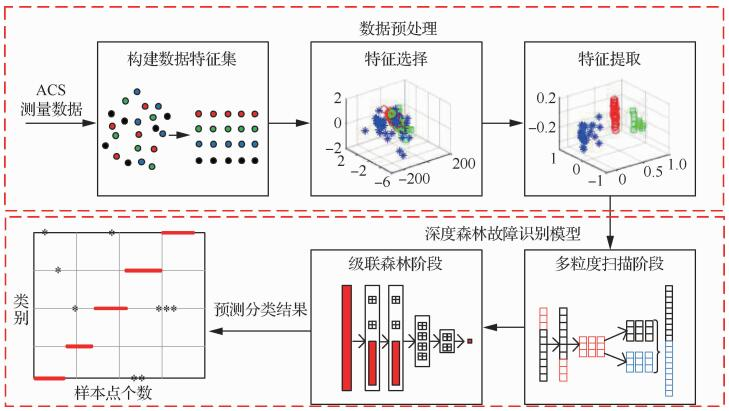图 2 卫星ACS故障识别策略 Fig. 2 Fault identification strategy of satellite attitude control systems
3 特征提取与识别方法 3.1 构建原始特征集

ACS飞轮采用无刷直流电机控制，其电机的力矩平衡方程为

 ${\mathit{\boldsymbol{M}}_{\rm{s}}} = {\mathit{\boldsymbol{J}}_{\rm{w}}}\frac{{{\rm{d}}{\mathit{\boldsymbol{\omega }}_{\rm{w}}}}}{{{\rm{d}}t}}$ （5）

 ${\mathit{\boldsymbol{M}}_{\rm{s}}} = \mathit{\boldsymbol{K}}\Delta {\mathit{\boldsymbol{n}}_{\rm{s}}}$ （6）

 ${\mathit{\boldsymbol{M}}_{\rm{c}}} = \mathit{\boldsymbol{K}}\Delta {\mathit{\boldsymbol{n}}_{\rm{c}}}$ （7）

 ${\mathit{\boldsymbol{M}}_{\rm{s}}} = {\mathit{\boldsymbol{M}}_{\rm{c}}} + {\mathit{\boldsymbol{M}}_{\rm{ \mathsf{ δ} }}} + {\mathit{\boldsymbol{f}}_{\rm{a}}}$ （8）

 $\Delta {\mathit{\boldsymbol{r}}_n} = \frac{1}{\mathit{\boldsymbol{K}}}({\mathit{\boldsymbol{M}}_{\rm{ \mathsf{ δ} }}} + {\mathit{\boldsymbol{f}}_{\rm{a}}})$ （9）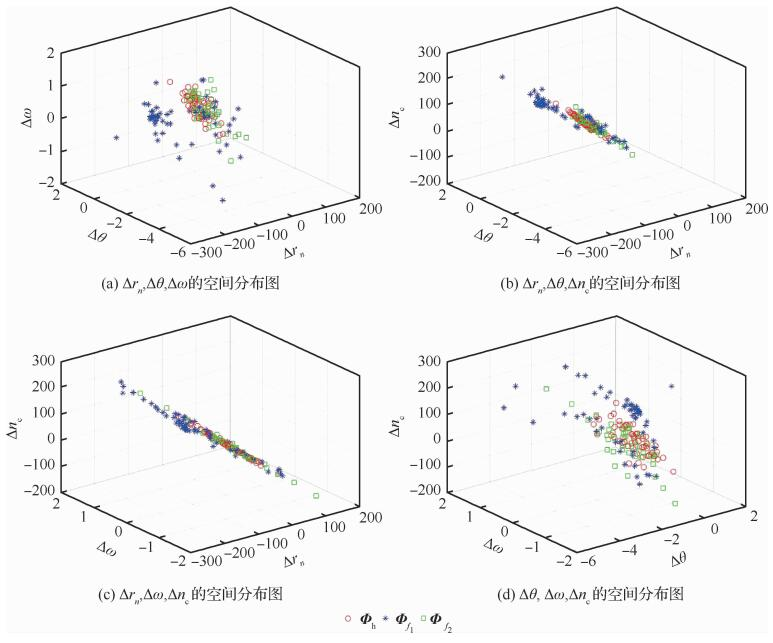图 3 Φf1、Φf2和Φh的原始特征点空间分布 Fig. 3 Spatial distribution of original feature points of Φf1, Φf2 and Φh
3.2 故障特征提取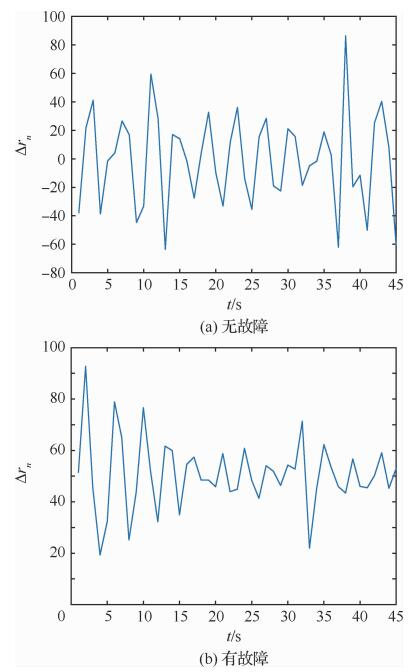图 4 Δrn的曲线图 Fig. 4 Graph of Δrn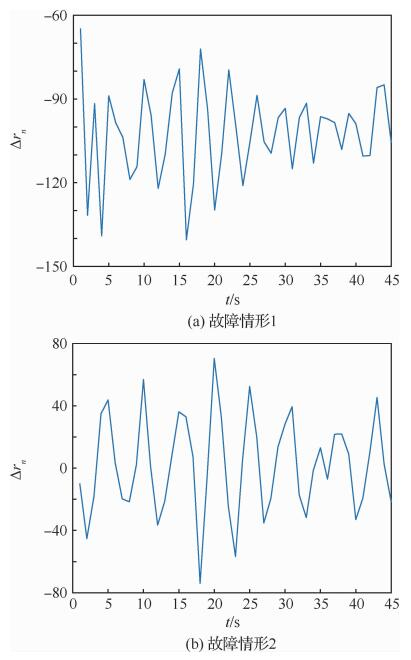图 5 不同故障情况下的Δrn曲线图 Fig. 5 Graphs of Δrn under different fault conditions

 $\left\{ \begin{array}{l} \mu = \frac{1}{N}\sum\limits_{i = 1}^N {{v_i}} \;\;i = 1,2, \cdots ,N\\ {\sigma ^2} = \frac{1}{{N - 1}}\sum\limits_{i = 1}^N {{{({v_i} - \mu )}^2}} \;\;i = 1,2, \cdots ,N\\ H = - \sum\limits_{k = 1}^c {{p_k}{{\log }_2}{p_k}\;\;k = 1,2, \cdots ,c} \\ I = \int {v(t){\rm{d}}t} \end{array} \right.$ （10）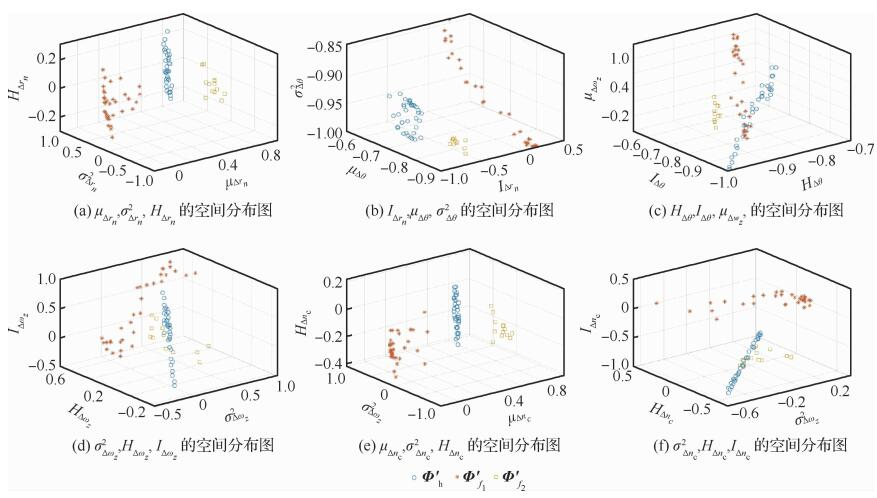图 6 Φ′f1、Φ′f2和Φ′h的特征点空间分布 Fig. 6 Spatial distribution of feature points of Φ′f1, Φ′f2 and Φ′h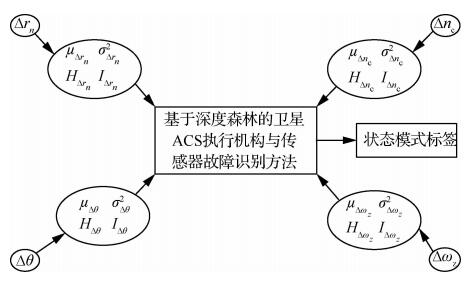图 7 基于深度森林的卫星ACS执行机构与传感器故障识别预测分类模型 Fig. 7 Fault recognition prediction and classification model of actuators and sensors in satellite ACS based on deep forest algorithm
3.3 深度森林故障识别方法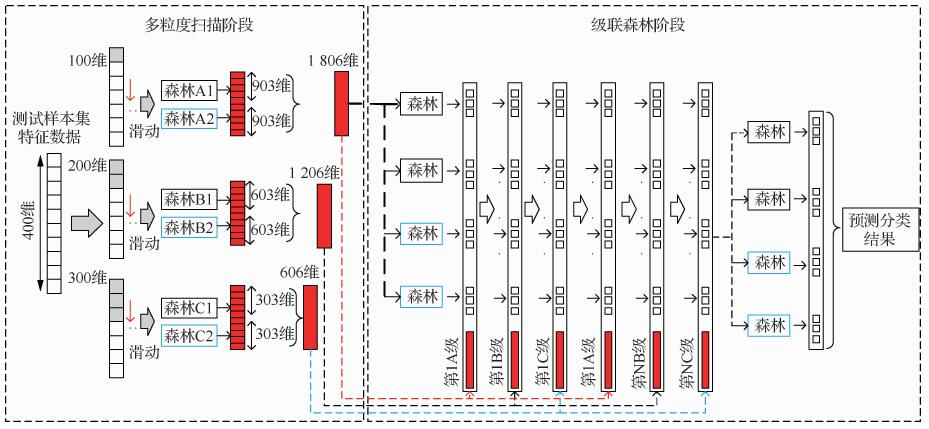图 8 深度森林算法整体预测过程 Fig. 8 Whole prediction process of deep forest algorithm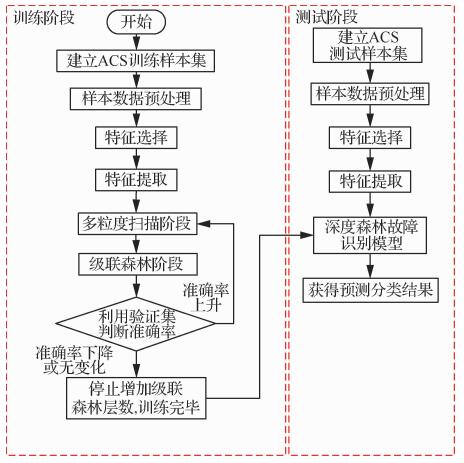图 9 基于深度森林的卫星ACS执行机构与传感器故障识别流程 Fig. 9 Flow chart of fault identification of sensor and actuator satellite ACS based on deep forest algorithm

1) 训练阶段

2) 测试阶段

4 仿真试验及结果分析 4.1 试验平台介绍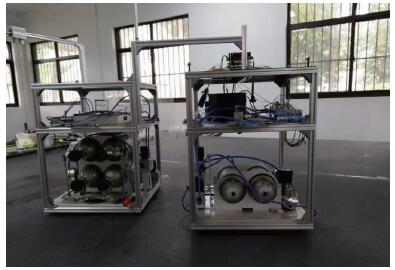图 10 某卫星半物理仿真平台示意图 Fig. 10 Schematic diagram of satellite semi-physical simulation platform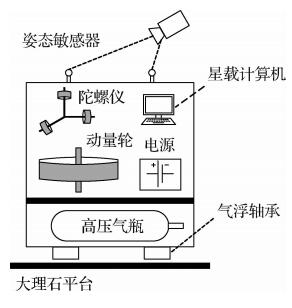图 11 某卫星半物理仿真平台系统组成示意图 Fig. 11 Composition of satellite semi-physical simulation platform system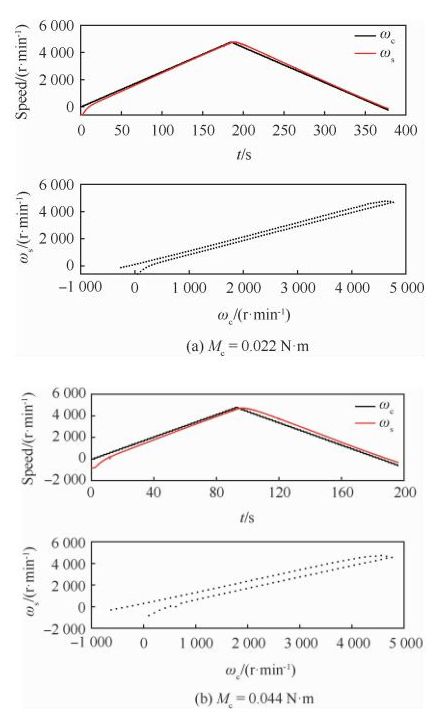图 12 不同指令力矩下的飞轮输出特性曲线 Fig. 12 Flywheel output characteristic curves under different command torques
4.2 故障模式设置

 故障类别 故障数学模型 故障原因 f1 $\begin{array}{l} {n_{\rm{o}}}(t) = \\ \left\{ {\begin{array}{*{20}{c}} {{n_{\rm{i}}}(t)}&{t < {t_{\rm{f}}}}\\ {{K_1}{n_{\rm{i}}}(t)}&{t \ge {t_{\rm{f}}}} \end{array}, {K_1} < 1} \right. \end{array}$ 驱动电路放大器故障 f2 $\begin{array}{l} {M_{\rm{o}}}(t) = \\ \left\{ {\begin{array}{*{20}{c}} {{M_{\rm{i}}}(t)}&{t < {t_{\rm{f}}}}\\ {{K_2}{M_{\rm{i}}}(t)}&{t \ge {t_{\rm{f}}}} \end{array}, {K_2} < 1} \right. \end{array}$ 驱动电机故障、由于温度的变化, 元器件老化等因素 f3 $\begin{array}{l} {M_{\rm{o}}}(t) = \\ \left\{ {\begin{array}{*{20}{c}} {{M_{\rm{i}}}(t)}&{t < {t_{\rm{f}}}}\\ {{M_{\rm{i}}}(t) + \delta (t)}&{{t_{\rm{f}}} \le t \le {t_{\rm{f}}} + \Delta t}\\ {{M_{\rm{i}}}(t)}&{t > {t_{\rm{f}}}} \end{array}} \right. \end{array}$ 总线电压故障或是电机存在间歇的时变故障 f4 $\begin{array}{l} {\omega _{\rm{o}}}(t) = \\ \left\{ {\begin{array}{*{20}{c}} {{\omega _{\rm{i}}}(t)}&{t < {t_{\rm{f}}}}\\ {{\omega _{\rm{i}}}(t) + {\omega _{\rm{f}}}(t)}&{t \ge {t_{\rm{f}}}} \end{array}} \right. \end{array}$ 陀螺线路盒电压输出不稳, 测量噪声增大 f5 $\begin{array}{l} {\theta _{\rm{o}}}(t) = \\ \left\{ {\begin{array}{*{20}{c}} {{\theta _{\rm{i}}}(t)}&{t < {t_{\rm{f}}}}\\ {{\theta _{\rm{i}}}(t) + {\theta _{\rm{f}}}(t)}&{t \ge {t_{\rm{f}}}} \end{array}} \right. \end{array}$ 存在干扰目标, CCD出现瑕疵点
4.3 故障定位结果及分析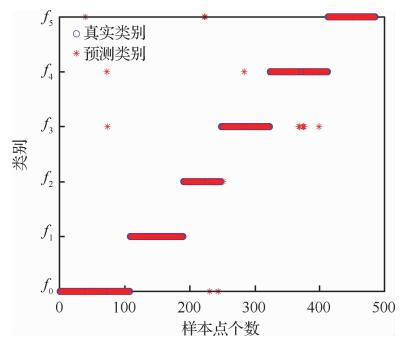图 13 深度森林故障识别模型对某一组测试集的故障识别结果 Fig. 13 Fault identification results of one test set of deep forest algorithm

 预测类别 识别准确率/% f0 f1 f2 f3 f4 f5 f0 98.15 0 0 1.23 0 0 f1 0 100 0 0 0 0 f2 0 0 96.55 0 0 0.42 f3 1.85 0 0 96.33 2.25 0.95 f4 0 0 1.65 1.09 97.75 0 f5 0 0 1.8 1.35 0 98.63

5 结论

  王巍, 宝音贺喜格, 姜兴渭, 等. 基于状态观测器的卫星执行机构故障诊断方法研究[J]. 黑龙江自动化技术与应用, 1999(4): 9-12. WANG W, BAOYIN H X G, JIANG X W, et al. Fault diagnosis method of actuators in satellite based on state observer[J]. Techniques of Automation and Applications, 1999(4): 9-12. (in Chinese) Cited By in Cnki (4) | Click to display the text  LI C L, CHEN X Q, JIA Q X, et al. FDD of the attitude control system of satellite with actuator fault and time delay via two-stage Kalman filter[C]//3rd International Conference on Machinery Electronics and Control Engineering, 2014, 441: 859-863.  江文建, 姜斌, 廖鹤, 等. 基于ILLE和SVM的卫星执行机构系统故障检测与定位[J]. 航天控制, 2019(3): 4. JIANG W J, JIANG B, LIAO H, et al. Fault detection and location of actuator system in satellite based on ILLE and SVM[J]. Aerospace Control, 2019(3): 4. (in Chinese) Cited By in Cnki (2) | Click to display the text  胡志坤, 孙岩, 姜斌, 等. 一种基于最优未知输入观测器的故障诊断方法[J]. 自动化学报, 2013, 39(8): 1225-1230. HU Z K, SUN Y, JIANG B, et al. An optimal unknown input observer based fault diagnosis method[J]. Acta Automatica Sinica, 2013, 39(8): 1225-1230. (in Chinese) Cited By in Cnki (60) | Click to display the text  樊雯, 程月华, 姜斌. 基于模糊滑模的卫星姿态控制系统故障诊断[J]. 东南大学学报(自然科学版), 2010, 40(S1): 238-243. FAN W, CHENG Y H, JIANG B. Fault diagnosis in attitude control system of satellite based on fuzzy sliding mode[J]. Journal of Southeast University (Natural Science Edition), 2010, 40(S1): 238-243. (in Chinese) Cited By in Cnki | Click to display the text  NADERI E, KHORASANI K. A data-driven approach to actuator and sensor fault detection, isolation and estimation in discrete-time linear systems[J]. Automatica, 2017, 85: 165-178. Click to display the text  WANG K, CHEN J, SONG Z. Concurrent fault detection and anomaly location in closed-loop dynamic systems with measured disturbances[J]. IEEE Transactions on Automation Science and Engineering, 2018. Click to display the text  CHENG Y, JIANG B, LU N, et al. Incremental locally linear embedding-based fault detection for satellite attitude control systems[J]. Journal of the Franklin Institute, 2016, 353(1): 17-36. Click to display the text  李磊, 高永明, 吴止锾, 等. 基于神经网络观测器的卫星姿态控制系统陀螺故障诊断[J]. 计算机测量与控制, 2018, 26(11): 7-11. LI L, GAO Y M, WU Z H, et al. Gyroscopic fault diagnosis method for satellite attitude control system based on neural network observer[J]. Computer Measurement & Control, 2018, 26(11): 7-11. (in Chinese) Cited By in Cnki | Click to display the text  MOUSAVI S, KHORASANI K. Fault detection of reaction wheels in attitude control subsystem of formation flying satellites:A dynamic neural network-based approach[J]. International Journal of Intelligent Unmanned Systems, 2014, 2(1): 2-26. Click to display the text  陈辛, 魏炳翌, 闻新. 基于支持向量机的卫星执行机构故障诊断研究[J]. 中国空间科学技术, 2018, 38(2): 47-55. CHEN X, WEI B Y, WEN X. Study of support vector machine based faults diagnosis for satellite's actuators[J]. Chinese Space Science and Technology, 2018, 38(2): 47-55. (in Chinese) Cited By in Cnki | Click to display the text  JEGADEESHWARAN R, SUGUMARAN V. Fault diagnosis of automobile hydraulic brake system using statistical features and support vector machines[J]. Mechanical Systems and Signal Processing, 2015, 52: 436-446. Click to display the text  CERRADA M, ZURITA G, CABRERA D, et al. Fault diagnosis in spur gears based on genetic algorithm and random forest[J]. Mechanical Systems and Signal Processing, 2016, 70: 87-103. Click to display the text  GRAVES A, MOHAMED A, HINTON G. Speech recognition with deep recurrent neural networks[C]//2013 IEEE International Conference on Acoustics, Speech and Signal Processing. Piscataway: IEEE Press, 2013: 6645-6649.  ZHOU Z H, FENG J. Deep forest:Towards an alternative to deep neural networks. arXiv 2017[J]. arXiv preprint arXiv:1702.08835. Click to display the text  陈吕鹏, 殷林飞, 余涛, 等. 基于深度森林算法的电力系统短期负荷预测[J]. 电力建设, 2018, 39(11): 42-50. CHEN L P, YIN L F, YU T, et al. Short-term power load forecasting based on deep forest algorithm[J]. Electric Power Construction, 2018, 39(11): 42-50. (in Chinese) Cited By in Cnki | Click to display the text  HU G, LI H, XIA Y, et al. A deep Boltzmann machine and multi-grained scanning forest ensemble collaborative method and its application to industrial fault diagnosis[J]. Computers in Industry, 2018, 100: 287-296. Click to display the text  沈文博, 孙荣霞, 马少卿, 等. 深度森林与人工神经网络在光伏出力预测的比较[J]. 信息技术与网络安全, 2018, 37(4): 49-51. SHEN W B, SUN R X, MA S Q, et al. Comparison of deep forest and artificial neural network in prediction of PV output[J]. Information Technology and Network Security, 2018, 37(4): 49-51. (in Chinese) Cited By in Cnki | Click to display the text  LI M, ZHANG N, PAN B, et al. Hyperspectral image classification based on deep forest and spectral-spatial cooperative feature[C]//International Conference on Image and Graphics. Berlin: Springer, 2017: 325-336.  UTKIN L V, KOVALEV M S, MELDO A A. A deep forest classifier with weights of class probability distribution subsets[J]. Knowledge-Based Systems, 2019, 173: 15-27. Click to display the text  康国华, 杨炳辉, 刘瑶, 等. 基于微小卫星编队的聚光操控技术[J]. 航天控制, 2018, 36(2): 42-47. KANG G H, YANG B H, LIU Y, et al. The technology of light control based on small satellite formation[J]. Aerospace Control, 2018, 36(2): 42-47. (in Chinese) Cited By in Cnki | Click to display the text
http://dx.doi.org/10.7527/S1000-6893.2019.23778

0

#### 文章信息

CHENG Yuehua, JIANG Wenjian, YANG Hao, XUE Qi, LIAO He

Fault identification of actuators and sensors of satellite attitude control systems based on deep forest algorithm

Acta Aeronautica et Astronautica Sinica, 2020, 41(S1): 723778.
http://dx.doi.org/10.7527/S1000-6893.2019.23778